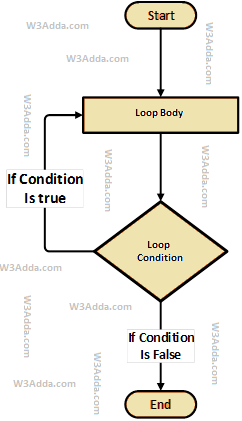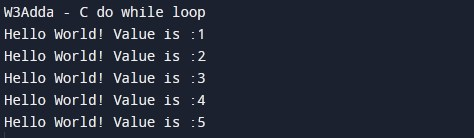# C Do While Loop

In this tutorial you will learn about the C Do While Loop and its application with practical example.

## C do-while Loop

In c programming, the do-while loop executes a block of statements inside the loop body first and then tests the condition. The next iteration executes only if the test condition is true.

The do-while loop is much similar to the while loop with one major difference, in the do-while loop block of statements inside the loop body executes at least once.

### Why We Need a do-while loop

The main difference between a while loop and a do-while loop is that a while loop is entry-controlled loop. On the other hand, the do-while loop is an exit-controlled loop. In a while loop, if the test condition becomes false in the first iteration then the loop body is not executed at all. But the do-while loop is somewhat different from the while loop. The do-while loop executes the loop body at least once even if the test condition is false for the first iteration. The do-while loop is mainly used where we want to execute the loop at least once.

### C do while Loop Flowchart

The following flowchart image illustrates the working of the do-while loop in C programming:### Do while Loop Syntax

Below is the general syntax of the do-while loop in c programming:

Syntax:-

Here, the loop executes a block of statements inside the loop body first and then tests the condition provided along with the while keyword for the next iteration and executes next only if the condition is true. Condition is a Boolean expression that results in either True or False, if it results in True then statements inside the loop body are executed and the condition is evaluated again. This process is repeated until the condition is evaluated as False. If the condition results in False then the loop is terminated and control is transferred to the next statement.

### Do while Loop Example

Below is a simple example to demonstrate the use of a do-while loop in c programming:

Example:-

Here, we have initialized ctr and maxCtr to 1 and 5 respectively, in the next statement we have loop body inside the pair of curly brackets {} after do keyword, statements inside loop body are executed first then control is passed to the condition ctr <= maxCtr provided along with while keyword for next iteration. If the test condition returns True, then control is passed again to the loop body for the next iteration. If the condition results in False then the loop is terminated and control is transferred to the next statement. Inside the loop body, we have incremented the ctr value by one for each iteration. When we run the above c program, we see the following output –

Output:-## Important Difference Between a while and do-while Loop

The main difference between a while loop and a do-while loop is that a while loop is an entry-controlled loop. On the other hand, the do-while loop is an exit-controlled loop. The while loop checks the test condition before executing the loop body. While the do-while loop checks the test condition after executing the statements inside the loop body. Here, we have listed some of the important Difference Between a while and do-while Loops.

# while do-while
Syntax while ( condition) {
statements; //body of loop
}
do{
.
statements; // body of loop.
.
} while( Condition );
Condition In the ‘while’ loop the test condition appears at the start of the loop. In the ‘do-while’ loop the test condition appears at the end of the loop.
Iterations The iterations do not occur if, the test condition for the first iteration is false. The iteration occurs at least once even if the test condition is false for the first iteration.
Type Entry-controlled loop Exit-controlled loop
Semi-colon Not used Used at the end of the loop

In this tutorial we have learn about the C Do While Loop and its application with practical example. I hope you will like this tutorial.